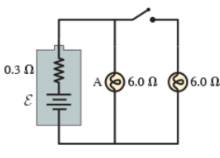# Problem: If the battery in (Figure 1) were ideal, lightbulb A would not dim when the switch is closed. However, real batteries have a small internal resistance, which we can model as the 0.3 Ω resistors shown inside the battery. In this case, the brightness of bulb A changes when the switch is closed. Assume ε = 2.0 VPart A. How much power does bulb A dissipate when the switch is open? Express your answer with the appropriate unitsPart B. How much power does bulb A dissipate when the switch is closed?  Express your answer with the appropriate units

###### FREE Expert Solution
94% (45 ratings)View Complete Written Solution
###### Problem Details

If the battery in (Figure 1) were ideal, lightbulb A would not dim when the switch is closed. However, real batteries have a small internal resistance, which we can model as the 0.3 Ω resistors shown inside the battery. In this case, the brightness of bulb A changes when the switch is closed. Assume ε = 2.0 VPart A. How much power does bulb A dissipate when the switch is open? Express your answer with the appropriate units

Part B. How much power does bulb A dissipate when the switch is closed?  Express your answer with the appropriate units